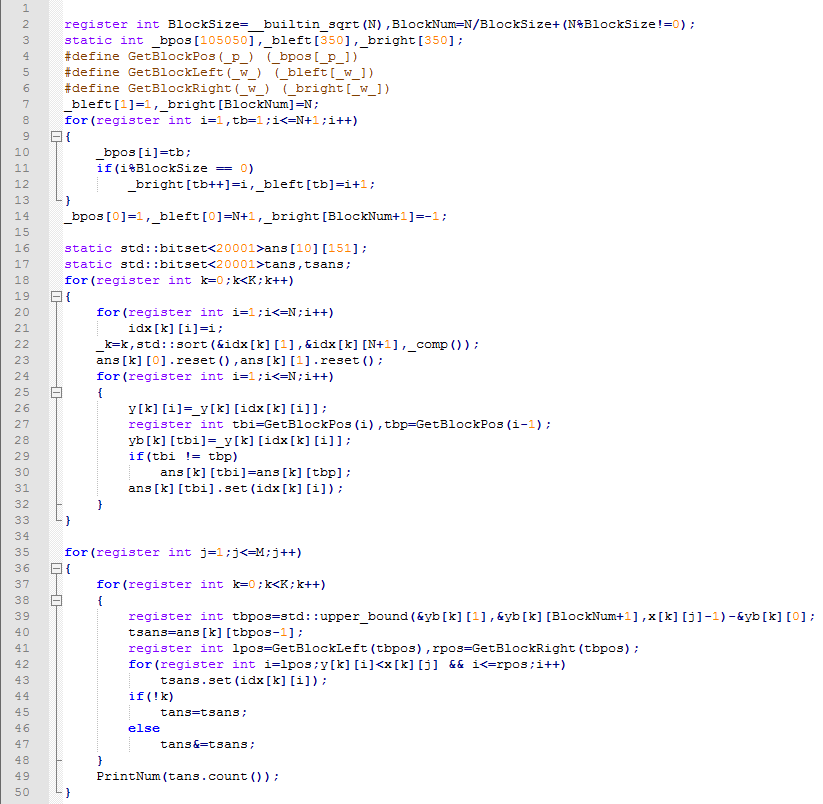# [研学] 偏序问题的研究

• ### 高维偏序…

# YZOJ P4444 [APIO2019]路灯

• ### 题目描述

$$toggle i$$：切换第 $$i$$ 个路灯的状态。具体地说，若路灯原来亮起，则现在将熄灭；若路灯原来熄灭，则现在将亮起。

$$query a b$$：自动驾驶的士部门的负责人想知道，从 $$0$$ 时刻起到当前时刻，有多少个时刻满足：自动驾驶的士能够从站点 $$a$$ 出发到达站点 $$b$$ 。

• ### 输入格式

$$toggle i$$ $$(1 \leq i \leq n)$$：该时刻切换了第 $$i$$ 个路灯的状态。

$$query a b$$ $$(1 \leq a < b \leq n+1)$$：计算从 $$0$$ 时刻起到该时刻，共有多少个时刻满足的士能从站点 $$a$$ 出发到达站点 $$b$$ 。

# YZOJ P2905 [PA2014]Druzyny

• ### 题目描述

OI组的神犇们决定把这题改造一下：

dick32165401：改成只有编号连续的的一段才可以分一组。

runzhe2000：判定可行解可能会被爆搜水过，最大化分的组数就不那么容易水过了。

E.Space：不仅要最大化组数，还要求出最大化组数的方案数。

ct：数据范围就出100万好了。

• ### 样例输出

Source: BZOJ 3711

# YZOJ P3924 [IOI2011]Race

• ### 题目描述

$$N \leq 200000, K \leq 1000000$$ 。

• ### 样例输出

Source: BZOJ 2599…

# YZOJ P3361 [校内训练20171117]数点问题

• ### 题目描述

$$k$$ 维空间内有两个点集 $$A=\{X_1,X_2,\ldots,X_m\}$$，$$B=\{Y_1,Y_2,\ldots,Y_n\}$$，每个点的坐标是一个 $$k$$ 元组 $$(x_1,x_2,\ldots,x_k)$$。我们称点 $$X(x_1,x_2,\ldots,x_k)$$ 控制点 $$Y(y_1,y_2,\ldots,y_k)$$ 当且仅当 $$\forall 1\le i\le k,x_i>y_i$$，记为 $$X>Y$$。数点问题是要求计算点 $$X_i$$ 能控制 $$B$$ 中的点数 $$c_i$$，即 $$c_i=\left| \{Y \in B\ |\ X_i > Y\} \right|$$。